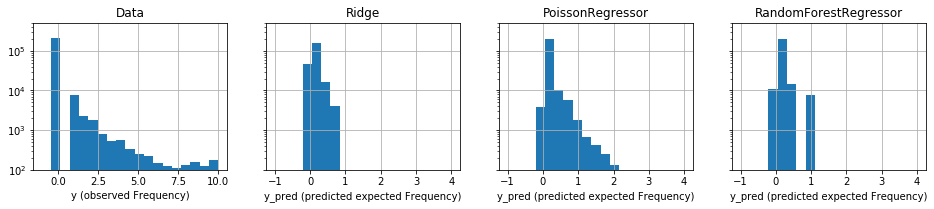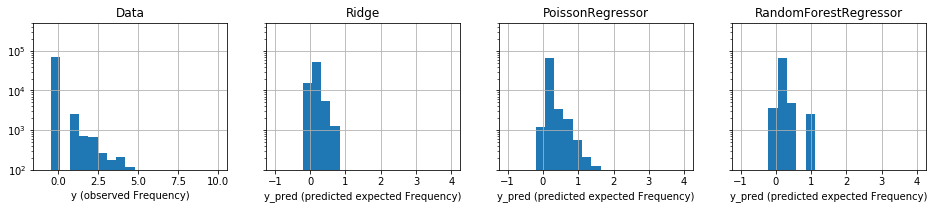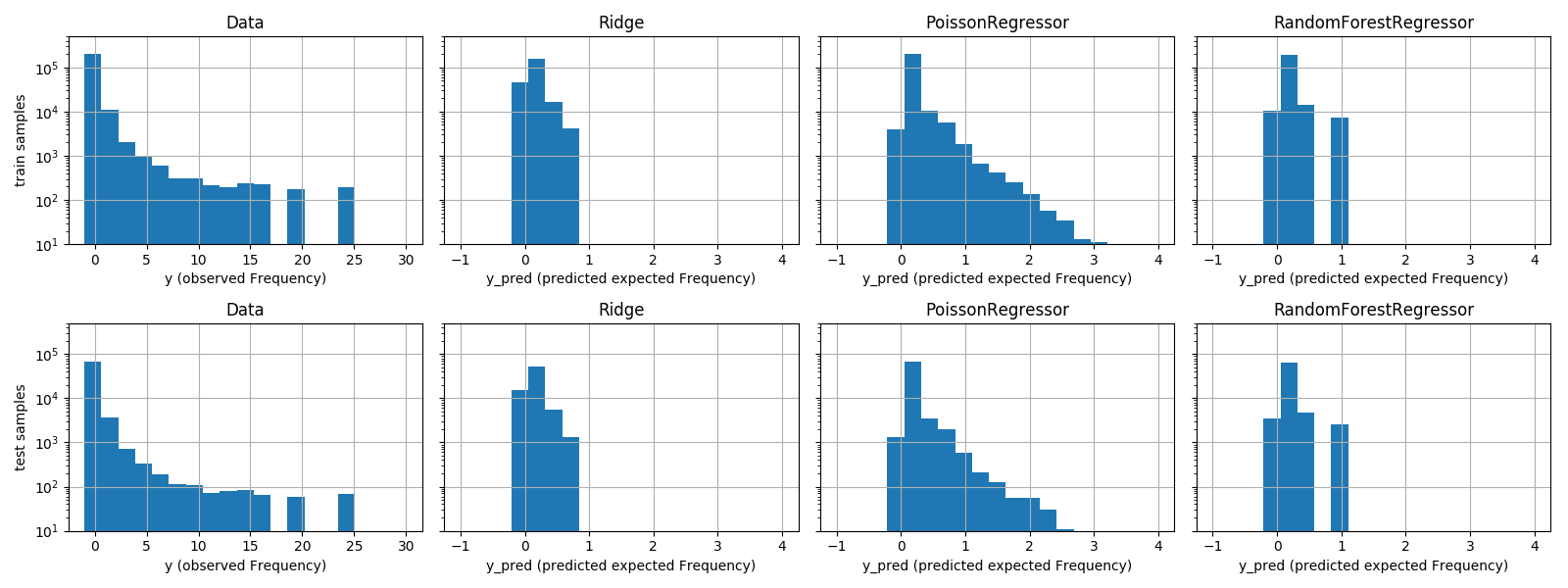scikit-learn/scikit-learn

Have a question about this project? Sign up for a free GitHub account to open an issue and contact its maintainers and the community.

By clicking “Sign up for GitHub”, you agree to our terms of service and privacy statement. We’ll occasionally send you account related emails.

Minimal Generalized linear models implementation (L2 + lbfgs) #14300

Open
wants to merge 223 commits into
from
+2,947 −54

Conversation

Member

rth commented Jul 9, 2019 • edited

A minimal implementation of Generalized Linear Models (GLM) from #9405 by @lorentzenchr

This only includes L2 penalty with the lbfgs solver. In other words, in excludes L1 penalties (or CD solver), matrix penalties, warm start, some distributions (e.g. BinomialDistribution), newton-cg & irls solvers from the original GLM PR.

The goals is to get an easier to review initial implementation. Benchmarks were done in #9405 (comment)

TODO

• fix user manual and comments regarding removed functionality
• examples may need some more work
• use a standalone Tweedie deviance metric #14263 once it is merged
• refactor the LBFS optimizer interface once #14250 is merged
• use a common function for sample_weight validations #14307
• expose objects that are for now not controversial to sklearn.linear_model
added 30 commits Jul 18, 2017
* Fixed pep8
* Fixed flake8
* Rename GeneralizedLinearModel as GeneralizedLinearRegressor
* Use of six.with_metaclass
* PEP257: summary should be on same line as quotes
* Docstring of class GeneralizedLinearRegressor: \ before mu
* Arguments family and link accept strings
* Use of ConvergenceWarning
* GeneralizedLinearRegressor added to doc/modules/classes.rst
* fixed bug: init parameter max_iter
* fix API for family and link:
default parameter changed to string
non public variables self._family_instance and self._link_instance
* fixed bug in score, minus sign forgotten
* added check_is_fitted to estimate_phi and score
* added check_array(X) in predict
* replaced lambda functions in TweedieDistribution
* some documentation
* make raw docstrings where appropriate
* make ExponentialDispersionModel (i.e. TweedieDistribution) pickable:
ExponentialDispersionModel has new properties include_lower_bound,
method in_y_range is not abstract anymore.
* set self.intercept_=0 if fit_intercept=False, such that it is always defined.
* set score to D2, a generalized R2 with deviance instead of squared error,
as does glmnet. This also solves issues with
check_regressors_train(GeneralizedLinearRegressor), which assumes R2 score.
* change of names: weight to weights in ExponentialDispersionModel and to
sample_weight in GeneralizedLinearRegressor
* add class method linear_predictor
* added L2 penalty
* api change: alpha, l1_ratio, P1, P2, warm_start, check_input, copy_X
* added entry in user guide
* improved docstrings
* helper function _irls_step
* fix some bugs in user guide linear_model.rst
* fix some pep8 issues in test_glm.py
* added test: ridge poisson with log-link compared to glmnet
* fix ValueError message for l1_ratio
* fix ValueError message for P2
* string comparison: use '==' and '!=' instead of 'is' and 'is not'
* fix RuntimeWarnings in unit_deviance of poisson: x*log(x) as xlogy
* added test for fisher matrix
* added test for family argument
* put arguments P1, P2 and check_input from fit to __init__
* added check_input test: is P2 positive definite?
* added solver option: 'auto'
* added coordinate descent solver
* skip doctest for GeneralizedLinearRegressor example
* symmetrize P2 => use P2 = 1/2 (P2+P2')
* better validation of parameter start_params
* bug for sparse matrices for newton-cg solver, function grad_hess
* reduce precision for solver newton-cg in test_poisson_ridge
* remedy doctest issues in linear_model.rst for example of GeneralizedLinearRegressor
* remove unused import of xrange from six
* bug in cd solver for sparse matrices
* higer precision (smaller tol) in test_normal_ridge for sparse matrices
* for each solver a separate precision (tol) in test_poisson_ridge
* improved documentation
* additional option 'zero' for argument start_params
* validation of sample_weight in function predict
* input validation of estimate_phi
* set default fit_dispersion=None
* bug in estimate_phi because of weight rescaling
* test for estimate_phi in normal ridge regression
* extended tests for elastic net poisson
* new helper function _check_weights for validation of sample_weight
* fix white space issue in doctest of linear_model.rst
* fit_dispersion default=None also in docs.
* improved docs.
* fixed input validation of predict
* fixed bug for sample_weight in estimate_phi
* improved docs
* fixed input validation of X in predict
* redundant line of code 'd = np.zeros_like(coef)'
* added test to compare to ElasticNet
* deleted identical comment lines
* increased precision in test_normal_enet
* better doc for heavy tailed distributions
* improved input validation and testing of them
* improved input validation and testing of P1
* test case for validation of argument P2
* test case for validation of argument copy_X
* fix doctest failure in example of linear_model.rst

* fix dtype issue in test_glm_P2_argument
* fix typos in doc
…spsolve about usage of umfpack
added 2 commits Oct 3, 2019
and others added 5 commits Oct 3, 2019
Co-Authored-By: Nicolas Hug <contact@nicolas-hug.com>
reviewed
Member

ogrisel left a comment

 A comment from a review that I started but I haven't completed yet:
 .. note:: * The feature matrix X should be standardized before fitting. This ensures that the penalty treats features equally.

ogrisel Oct 4, 2019

Member

Standardized might be problematic if:

• one expects non-linear threshold effects in which case binning might be interesting;
• one expects heavy tailed marginal distribution in which case PowerTransformer or QuantileTransformer might be more suitable.

Maybe one could just say "scaled before fitting to ensure that the penalty treats features approximately equally"

added 2 commits Oct 6, 2019
Contributor

lorentzenchr commented Oct 6, 2019 • edited

What I do not understand however is the larger discrepancy in the y_pred histograms of the two models:
...
How can it the RF model be well calibrated for the top 10% of the high risk policy holders while having so few large y_pred values (non above 1.07)?

@ogrisel @rth Very interesting/hard questions about the Poisson Example!
First, the plot 'Mean Frequency (y_pred)' is on the test set and indicates good calibration of RF and Poisson, as you said. Note, that in all bins (x axis) the average frequency is below 0.6 (y axis).

Second, the histograms are on the training set and have different scales on y-axis. A changed that with sharey=True:Same on the test set:Note that the distribution of y_pred (1st column) visually reaches 10 in the training and 5 in the test set. Furthermore, the Poisson model always has highest predicted values, around 2, while the RF has max prediction around 1.

Third, evaluating the Poisson deviance on observations with y_true==0 and y_true>0 separately on the test set gives:

sample Poisson RF
y_true=0 0.254 0.254
y_true>0 5.795 5.685

This indicates, that the most part of the difference in Poisson deviance comes from y_true > 0.

I draw the following conclusions/hypothesis:

1. In feature space, the average frequency is by far lower than the maximal observed values of single observations (also for the top 10% riskiest policies). That's why RF is well calibrated and predicts maximal around 1. And it fits well to the assumption of an overlap of different Poisson distributions with different mean parameter for the target.

2. The test sample has less extreme values, which favours models that predict less extreme. (Maybe investigate with CV.)

3. One would expect the RF with squared error to predict more extreme values, but it seems that the non-parametric approach outweighs this shortcoming. The RF even has no prediction around 0.75, i.e. it finds a gap in feature space.

4. The Poisson model is presumably forced to predict large values due to an insufficient feature preprocessing. Especially continuous features like 'BonusMalus' and 'Density' might cause this.

Member

ogrisel commented Oct 8, 2019

 First, the plot 'Mean Frequency (y_pred)' is on the test set and indicates good calibration of RF and Poisson, as you said. Note, that in all bins (x axis) the average frequency is below 0.6 (y axis). That's a good point. By increasing the number of bins (e.g n_bins>=100) in this plot, one can see that the linear Poisson model can indeed predict larger y_pred values for the extreme bin than the RF model would and on this test set those extreme predictions tend to be too large. However the plots tend to be too noisy and less readable so I prefer to keep n_bins=10 for the example. But the (suboptimal) behavior of the linear Poisson model in the most risky percentile is probably what explains this counter-intuitive difference on this histograms. Also the fact that y-axis of the histogram is in log-scale is over-emphasizing the fraction of the samples with large y_pred. The test sample has less extreme values, which favours models that predict less extreme. (Maybe investigate with CV.) The test samples actually have approximately the same distribution (random shuffle split with hundreds of thousands of samples). The test set is smaller and the ylim threshold at 1e2 would hide the extreme values:I will push a fix for this. I tried to change the random_state of the split, the plots look qualitatively the same although the performance metrics change quite a bit although they stay in the same relative order. A few extreme samples probably have a significant impact on the perf metrics but all models seem to make correlated errors, so the conclusions are invariant to the random split from a model selection point of view. One would expect the RF with squared error to predict more extreme values, but it seems that the non-parametric approach outweighs this shortcoming. The RF even has no prediction around 0.75, i.e. it finds a gap in feature space. It would be interesting to explore the "learned representation" of the RF model by projecting the data into a one-hot encoded high dim binary code with one dimension per leaf in the RF and then use PCA or PCA+t-SNE/UMAP to visualize the distribution of the test set any introspect the nature of the cluster identified by the RF model and how they relate to the risky-ness of the policyholders. But this example is already complex enough like this I think. The Poisson model is presumably forced to predict large values due to an insufficient feature preprocessing. Especially continuous features like 'BonusMalus' and 'Density' might cause this. Interesting hypothesis. I have tried binning those features but it did not change much the results (slightly worse deviance, although probably not significant) and similar histogram for y_pred. But maybe the model would need to capture feature interactions to avoid having to predict large values.
and others added 4 commits Oct 8, 2019
reviewed
Member

thomasjpfan left a comment

 First pass
doc/modules/linear_model.rst Outdated Show resolved Hide resolved
 class ExponentialDispersionModel(metaclass=ABCMeta): r"""Base class for reproductive Exponential Dispersion Models (EDM). The pdf of :math:Y\sim \mathrm{EDM}(y_\textrm{pred}, \phi) is given by

thomasjpfan Oct 9, 2019

Member

Are we okay with having math in the docstring?

rth Oct 10, 2019

Author Member

See discussion in #14300 (comment)

 y_pred : array, shape (n_samples,) Predicted mean. """ pass # pragma: no cover

thomasjpfan Oct 9, 2019

Member

Nit: Does this work?

Suggested change
 pass # pragma: no cover ...

rth Oct 10, 2019

Author Member

Does this work?

Nope, it doesn't.

thomasjpfan Oct 10, 2019

Member

From my understanding, we can completely remove the body for abstract methods. This is similar to what we do in LossFunction:

Lines 43 to 58 in 9d5dd3e

 @abstractmethod def __call__(self, y, raw_predictions, sample_weight=None): """Compute the loss. Parameters ---------- y : 1d array, shape (n_samples,) True labels. raw_predictions : 2d array, shape (n_samples, K) The raw predictions (i.e. values from the tree leaves). sample_weight : 1d array, shape (n_samples,), optional Sample weights. """

 copy_X=copy_X, verbose=verbose) @property def family(self):

thomasjpfan Oct 9, 2019

Member

Should the family property be private?

 """ return weights * self.unit_deviance_derivative(y, y_pred) def _y_pred_deviance_derivative(self, coef, X, y, weights, link):

thomasjpfan Oct 9, 2019

Member

Does this need to be an instance method or is a private function enough for our use case?

(For our use case, we are calling this in the function passed to the lbfgs solver.)

Show resolved Hide resolved
Show resolved Hide resolved
sklearn/linear_model/_glm/glm.py Outdated Show resolved Hide resolved
sklearn/linear_model/_glm/glm.py Outdated Show resolved Hide resolved
 added to the linear predictor (X*coef+intercept). family : {'normal', 'poisson', 'gamma', 'inverse.gaussian'} \ or an instance of class ExponentialDispersionModel, \

thomasjpfan Oct 9, 2019

Member

I am okay with this since GeneralizedLinearRegressor is not public.

reviewed
 search = GridSearchCV( glm_total, cv=3, param_grid=params, n_jobs=-1, verbose=10, refit=False,

thomasjpfan Oct 9, 2019

Member

This gridsearch is still using r2 for its scoring, but the description says we are using grid search to minimize deviance.

There may not be a nice way to do this, because we are searching over power, the mean_tweedie_deviance metric depends on power.

ogrisel Oct 9, 2019

Member

Grid search is maximizing D2 (explained deviance ratio) which is the same as minimizing deviance which itself depends on power...

thomasjpfan Oct 9, 2019

Member

Ahh I see that the score is overwritten for this regressor.

NicolasHug Oct 9, 2019

Contributor

I am confused here: isn't GridSearch going to compare scores that are not comparable?

est.score depends on the power and the power is being searched over.

ogrisel Oct 10, 2019

Member

@NicolasHug I share your concern. I am not sure if choosing the value of power that maximizes D^2 is a methodological error or not. @lorentzenchr @agramfort what is your opinion?

For reference here is the meaning of the deviance of a GLM:

NicolasHug Oct 10, 2019

Contributor

using grid search over values of p (particularly for the compound Poisson Gamma (1 < p < 2) might be OK

what about when p leads to completely different distributions? e.g. the unit deviance for normal is
dev = (y - y_pred)**2
vs poisson
dev = 2 * (xlogy(y, y/y_pred) - y + y_pred)

Are these really comparable? I didn't plot them but I wouldn't be surprised if the normal was consistently higher. The selected p would always come from the normal.

lorentzenchr Oct 10, 2019

Contributor

Thanks @rth for the citations and @ogrisel for your many contributions lately. I'll try to further clarify my point of view:

• For model fitting, we could fully specify a stochastic model and obtain all parameters by maximum likelihood, i.e. not only coef_ but also the power parameter p. The deviance is a part of the log-likelihood. Unfortunately, it does not contain all dependency on p (but all that is needed for the expected value). Therefore, we should not use a grid search to find an optimal value for p based on deviance, that depends on this same p. We should set it a priori. One could even fit two models, one with, say, p=1.5 and another with p=1.9.
• For model comparison with a deviance, I strongly recommend to set power in advance or use several metrics, say, one with p=1.5, another with p=1.9. Other metrics like MAE are not suitable here, because they elicit the median (or something else), but not the expected value (conditional on X), which is our goal.
• D2 is equivalent to mean_tweedie_deviance with the same power parameter when comparing models.
• Wanna use GridSearch: One could specify a metric a priori, say power=1.5 and use this metric to find the best value of p via CV. I expect to find power≈p, but this may also depend on other hyperparameters, mostly the penalty.

main message: distinction between loss (training, p) and metric (model comparison on test/validation data, power)

ogrisel Oct 11, 2019 • edited

Member

Thanks for you feedback @lorentzenchr. In #15176 I have updated the example to use the Gini index as a model selection metric in the grid search. It also selects power=2.

Wanna use GridSearch: One could specify a metric a priori, say power=1.5 and use this metric to find the best value of p via CV. I expect to find power≈p, but this may also depend on other hyperparameters, mostly the penalty.

That makes sense, I think we should try to implement this in the example. I also expect the feature engineering to have a potential impact.

NicolasHug Oct 11, 2019

Contributor

we should not use a grid search to find an optimal value for p based on deviance

So since est.score is the deviance, we should not allow users to grid search on p? I don't think we have an API for forbidding users to grid search on something

ogrisel Oct 11, 2019

Member

No need for forbidding. Proper documentation and examples are enough in my opinion.

and others added 8 commits Oct 9, 2019
Co-Authored-By: Thomas J Fan <thomasjpfan@gmail.com>
to join this conversation on GitHub. Already have an account? Sign in to comment
Projects
None yet
You can’t perform that action at this time.